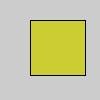Name

### & (bitwise AND)

Examples```a = 207    # In binary: 11001111
b = 61     # In binary: 00111101
c = a & b  # In binary: 00001101
print(c) # Prints "13", the decimal equivalent to 00001101
``````argb = color(204, 204, 51, 255)
# The sytax "& 0xFF" compares the binary
# representation of the two values and
# makes all but the last 8 bits into a 0.
# "0xFF" is 00000000000000000000000011111111
a = argb >> 24 & 0xFF
r = argb >> 16 & 0xFF
g = argb >> 8 & 0xFF
b = argb & 0xFF
fill(r, g, b, a)
rect(30, 20, 55, 55)
```
Description Compares each corresponding bit in the binary representation of the values. For each comparison two 1's yield 1, 1 and 0 yield 0, and two 0's yield 0. This is easy to see when we look at the binary representation of numbers

```  11010110  # 214
& 01011100  # 92
--------
01010100  # 84```

To see the binary representation of a number, use the binary() function with print().
Syntax
`value & value2`
Parameters
value1 int, char, byte int, char, byte
Related | (bitwise OR)
binary()
Updated on Fri Aug 16 21:51:57 2019.